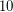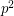# prime

## Sum of prime and semiprime conjecture ★★

Author(s): Geoffrey Marnell

Conjecture   Every even number greater thancan be represented as the sum of an odd prime number and an odd semiprime .

Keywords: prime; semiprime

## Polignac's Conjecture ★★★

Author(s): de Polignac

Conjecture   Polignac's Conjecture: For any positive even number n, there are infinitely many prime gaps of size n. In other words: There are infinitely many cases of two consecutive prime numbers with difference n.

In particular, this implies:

Conjecture   Twin Prime Conjecture: There are an infinite number of twin primes.

Keywords: prime; prime gap

## Are there an infinite number of lucky primes? ★

Author(s): Lazarus: Gardiner: Metropolis; Ulam

Conjecture   If every second positive integer except 2 is remaining, then every third remaining integer except 3, then every fourth remaining integer etc. , an infinite number of the remaining integers are prime.

Keywords: lucky; prime; seive

## Twin prime conjecture ★★★★

Author(s):

Conjecture   There exist infinitely many positive integersso that bothandare prime.

Keywords: prime; twin prime

## Wall-Sun-Sun primes and Fibonacci divisibility ★★

Author(s):

Conjecture   For any prime, there exists a Fibonacci number divisible byexactly once.

Equivalently:

Conjecture   For any prime,does not dividewhereis the Legendre symbol.

Keywords: Fibonacci; prime

## Goldbach conjecture ★★★★

Author(s): Goldbach

Conjecture   Every even integer greater than 2 is the sum of two primes.